# Python: Introduction IIIThe last part of the Python Introduction and I will cover topics on functions, methods and the packages in Python. For sure, there is a difference between function and method. I revisit my original post which I wrote about the differences between functions and methods. You can read up those before continue here.

## User-defined Functions

The simplest way I can explain what is function which I wrote in my original post:

A function is a block of code to carry out a task and it calls by its name. All functions may have zero or many arguments. The arguments are passed explicitly (directly). On the exit of the function, it may or may not return value or values.

There are some examples in this post to explain about functions, how to define a function with and without arguments, uses default value for an argument, uses flexible arguments *args and **kwargs and uses of return statement in the function.

## Methods

It is like a function, except it is attached to an object (dependent). A method is implicitly (indirectly) passed to the object for which it is invoked. It may or may not return a value or values. The method is accessible to data that is contained within the class.

For methods examples, I wrote it in this post.

## Packages

Think the packages as a directory of Python scripts. Example each .py script is a module. This module specifies functions, methods and types in solving a particular problem. I found a link which explained in detail about packages in Python. Refer here for more reading.

In this part III, I know there are many external links are given, mainly is to reduce re-write of those entries which I wrote them sometimes ago. This blog serves as a place to find the relevant resources for reading and examples which I think it is enough to cover the basic understanding of the functions, methods and packages in Python.

# World Bank World Development Indicator Case Study with PythonIn the DataCamp’s tutorials, Python Data Science Toolbox (Part 2), it combines user defined functions, iterators, list comprehensions and generators to wrangle and extract meaningful information from the real-world case study.

It is going to use World Bank’s dataset. The tutorials will use all which I have learned recently to work around with this dataset.

Dictionaries for Data Science

The zip() function to combine two lists into a zip object and convert it into a dictionary.

Before I share the sample code, let me share again what the zip() function does.

Using zip()
It allows us to stitch together any arbitrary number of iterables. In other words, it is zipping them together to create a zip object which is an iterator of tuple.

```# Zip lists: zipped_lists
zipped_lists = zip(feature_names, row_vals)

# Create a dictionary: rs_dict
rs_dict = dict(zipped_lists)

# Print the dictionary
print(rs_dict)

# Output: {'CountryName': 'Arab World', 'CountryCode': 'ARB', 'IndicatorName': 'Adolescent fertility rate (births per 1,000 women ages 15-19)', 'IndicatorCode': 'SP.ADO.TFRT', 'Year': '1960', 'Value': '133.56090740552298'}
```

Next, the tutorial wants us to create an user defined function with two parameters. I can re-use the above code and add an user defined function and call it with passing two arguments, feature_names and row_vals.

```# Define lists2dict()
def lists2dict(list1, list2):
"""Return a dictionary where list1 provides
the keys and list2 provides the values."""

# Zip lists: zipped_lists
zipped_lists = zip(list1, list2)

# Create a dictionary: rs_dict
rs_dict = dict(zipped_lists)

# Return the dictionary
return rs_dict

# Call lists2dict: rs_fxn
rs_fxn = lists2dict(feature_names, row_vals)

# Print rs_fxn
print(rs_fxn)
```

It should give the same result when run the codes. Next, tutorial requires me to use list comprehension. It requires to turn a bunch of lists into a list of dictionaries with the help of a list comprehension, where the keys are the header names and the values are the row entries.

The syntax,
`[[output expression] for` `iterator variable in` `iterable]`

The question in the tutorial is,
Create a list comprehension that generates a dictionary using lists2dict() for each sublist in row_lists. The keys are from the feature_names list and the values are the row entries in row_lists. Use sublist as your iterator variable and assign the resulting list of dictionaries to list_of_dicts.

The code on the screen before I coded. As above, sublist is the iterator variable, so it is substituted in between “for” and “in” keyword. The instruction says,
for each sublist in row_lists

indirectly, it means,

```# Turn list of lists into list of dicts: list_of_dicts
list_of_dicts = [--- for sublist in row_lists]
```

The lists2dict() function which I created above returns a dictionary. The question says,
generates a dictionary using lists2dict()

indirectly it means calling the lists2dict() function at the output expression. But if I code,

```# Turn list of lists into list of dicts: list_of_dicts
list_of_dicts = [lists2dict(feature_names, row_lists) for sublist in row_lists]
```

The output was very wrong and when I clicked the “Submit” button, it prompted me error message,
Check your call of lists2dict(). Did you correctly specify the second argument? Expected sublist, but got row_lists.

It expected sublist and yes, the for loop is reading each list in the row_lists. I have a code to print each list,
print(row_lists)

It is more meaningful to use sublist as 2nd argument rather than using row_lists. Therefore, the final code is,

```# Print the first two lists in row_lists
print(row_lists)
print(row_lists)

# Turn list of lists into list of dicts: list_of_dicts
list_of_dicts = [lists2dict(feature_names, sublist) for sublist in row_lists]

# Print the first two dictionaries in list_of_dicts
print(list_of_dicts)
print(list_of_dicts)
```
```#Output:
{'CountryName': 'Arab World', 'CountryCode': 'ARB', 'IndicatorName': 'Adolescent fertility rate (births per 1,000 women ages 15-19)', 'IndicatorCode': 'SP.ADO.TFRT', 'Year': '1960', 'Value': '133.56090740552298'}
{'CountryName': 'Arab World', 'CountryCode': 'ARB', 'IndicatorName': 'Age dependency ratio (% of working-age population)', 'IndicatorCode': 'SP.POP.DPND', 'Year': '1960', 'Value': '87.7976011532547'}

```

The above code is really taking my time to find out what I should code and why it did not get a right code. That did not stop me from continuing my tutorial.

Turning it into a DataFrame
Up to here, this case study I did a zip() function, put it into an user defined function and used the new created function in list comprehensions to generate a list of dictionaries.

Next, the tutorial wants to convert the list of dictionaries into Pandas’ DataFrame. First and foremost, I need to import the Pandas package. Let refer to the code below:

```# Import the pandas package
import pandas as pd

# Turn list of lists into list of dicts: list_of_dicts
list_of_dicts = [lists2dict(feature_names, sublist) for sublist in row_lists]

# Turn list of dicts into a DataFrame: df
df = pd.DataFrame(list_of_dicts)

# Print the head of the DataFrame

```

Summary of the day:

• zip() function combines the lists into a zip object.
• Use user defined function in list comprehension.
• Convert list comprehension into a DataFrame.

# Day 39: Using Iterator for Big Data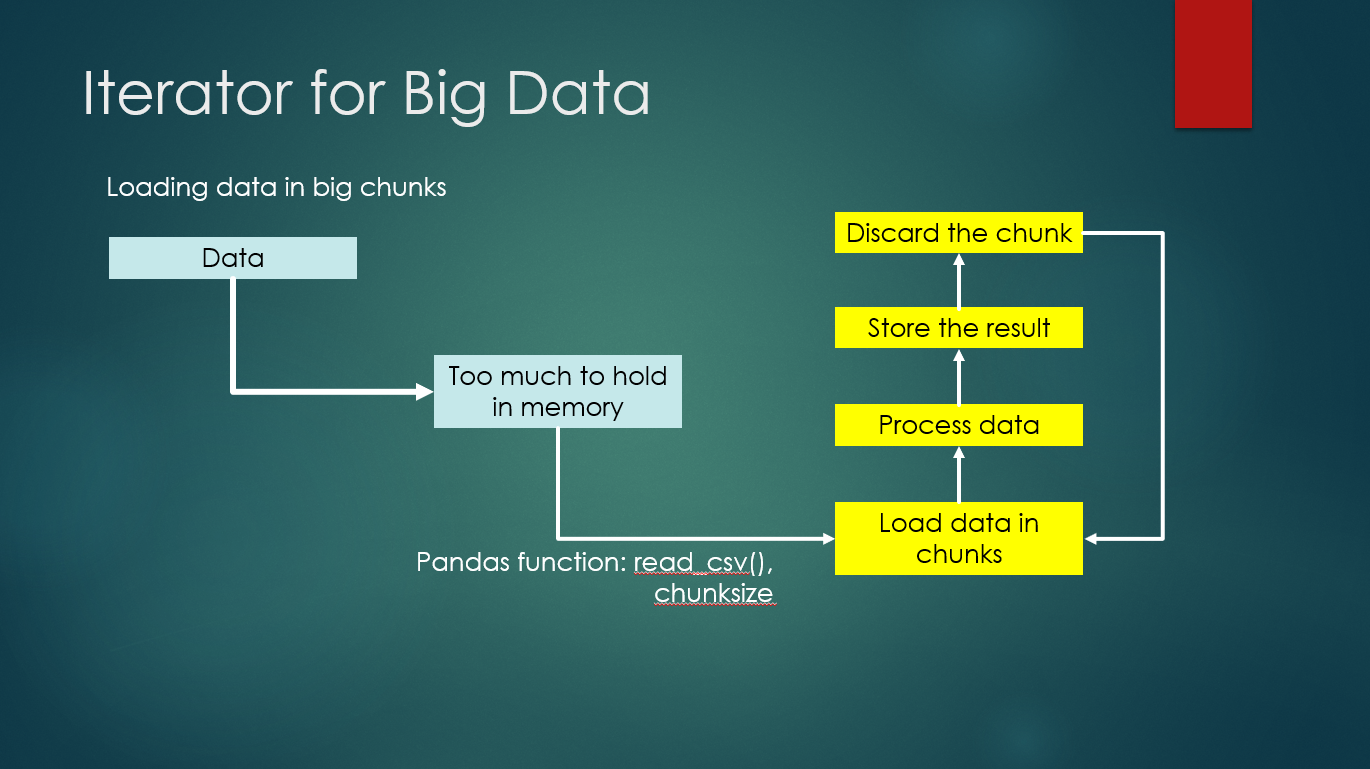The above illustrate the real scenario of data science where often they need to load a big chunk of data and sometimes it is too huge to be handled by the memory. The usage of Pandas, read_csv() function and setting the chunksize, it helps to load data in a smaller chunk, process the data and store the result somewhere before discard the chunk to load the next set to be processed. This is where iterator becomes useful.

Examples as below: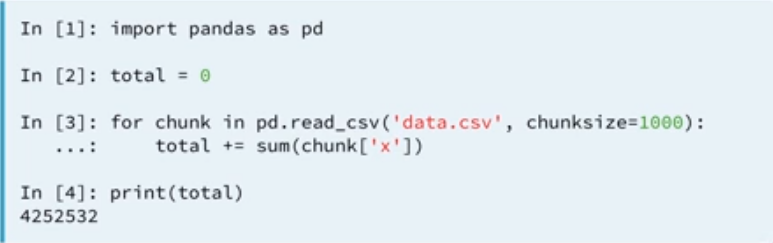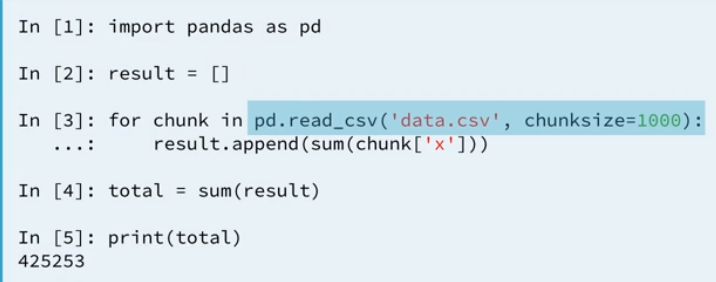Either we use a variable “total” to hold the sum’s result or we can create an empty dictionary to perform the same computation and it gives the same result. Below is the exercise I did in DataCamp’s online learning website using the Twitter’s data.

```# Initialize an empty dictionary: counts_dict
counts_dict = {}

# Iterate over the file chunk by chunk

# Iterate over the column in DataFrame
for entry in chunk['lang']:
if entry in counts_dict.keys():
counts_dict[entry] += 1
else:
counts_dict[entry] = 1

# Print the populated dictionary
print(counts_dict)
```

Create an empty dictionary, iterate over the csv file with chunksize is 10. Read the ‘lang’ column in the chunk and iterate again to get the count of each ‘lang’ in the .csv file. The output on the screen when executed is:

{‘en’: 97, ‘et’: 1, ‘und’: 2}

Let us convert the above code into an user defined function and takes three parameters, the csv filename, the chunk size and column name in the csv file. The updated version of the code with user defined function looks as below:

```# Define count_entries()
def count_entries(csv_file, c_size, colname):
"""Return a dictionary with counts of
occurrences as value for each key."""

# Initialize an empty dictionary: counts_dict
counts_dict = {}

# Iterate over the file chunk by chunk

# Iterate over the column in DataFrame
for entry in chunk[colname]:
if entry in counts_dict.keys():
counts_dict[entry] += 1
else:
counts_dict[entry] = 1

# Return counts_dict
return counts_dict

# Call count_entries(): result_counts
result_counts = count_entries('tweets.csv', 10, 'lang')

# Print result_counts
print(result_counts)
```

It gives the same result as the previous code.

Summary of the day:

• Iterator for Big Data.
• Using dictionaries, for loop statement to iterate data.
• Create an user defined function for the above and call to the function to print out result.

# Day 36: Lambda Functions in PythonAfter mastering how to write our own functions, we can quickly write functions on the fly by using the keyword, lambda.

The syntax is,
lambda value1, value2 : expression

Main keywords for the above syntax:
lambda: to indicate it is a shorthand of a function declaration.
argument: value1, value2, etc are the names of arguments.
colon (:): to indicate beginning of the expression.
expression: specifies what we wish the function to return.

Lambda functions allow us to write functions in quick and simplified way. However, it is not advisable to use it all the time.

Sample code:

```raise_to_power = lambda x, y: x ** y
raise_to_power(2,3)
```

Another example extracted from my exercise in DataCamp:

```# Define echo_word as a lambda function: echo_word
echo_word = (lambda word1, echo: word1 * echo)

# Call echo_word: result
result = echo_word('hey', 5)

# Print result
print(result)

#Output: heyheyheyheyhey
```

If you run the above code at the Jupyter Notebook’s online, the output is,
heyheyheyheyhey

To understand the idea of using lambda function, let us try to use the map() function.

map() function applies a function over an object such as a list. Use the lambda function to define the function that map() will use to process the object. See sample code below:

```nums = [2,4,6,8,10]
result = map(lambda a: a ** 2, nums)

#Convert into a list and print
list_result = list(result)
print(list_result)

#Output: [4, 16, 36, 64, 100]
```

The nums is a list. The map object that results from the call to map() is stored in the variable result. What you can do next is convert the map object into a list and print out the value. How to do so?

The line of code below converts map object into a list,
list_result = list(result)

and you can see the output of executing the above code, all the numbers in the nums list are power by 2. In another simple example of using map() function with lambda,

```# Create a list of strings: spells
spells = ["protego", "accio", "expecto patronum", "legilimens"]

# Use map() to apply a lambda function over spells: shout_spells
shout_spells = map(lambda item: item + '!!!', spells)

# Convert shout_spells to a list: shout_spells_list
shout_spells_list = list(shout_spells)

# Convert shout_spells into a list and print it
print(shout_spells_list)
```

By now, you will know what the output is. All items in the lists are concatenated with ‘!!!”. Now we try something new!

Below exercise extracted from DataCamp, I was trying to use a lambda function with filter() function. This filter() function offers a way to filter out elements from a list that do not satisfy certain criteria.

The question of the exercise:
In the filter() call, pass a lambda function and the list of strings, fellowship. The lambda function should check if the number of characters in a string member is greater than 6; use the len() function to do this. Assign the resulting filter object to result. Convert result to a list and print out the list.

```# Create a list of strings: fellowship
fellowship = ['frodo', 'samwise', 'merry', 'pippin', 'aragorn', 'boromir', 'legolas', 'gimli', 'gandalf']

# Use filter() to apply a lambda function over fellowship: result
result = filter(lambda member: len(member) > 6, fellowship)

# Convert result to a list: result_list
result_list = list(result)

# Convert result into a list and print it
print(result_list)

#Output: ['samwise', 'aragorn', 'boromir', 'legolas', 'gandalf']
```

The reduce() function is useful for performing some computation on a list and, unlike map() and filter(), returns a single value as a result. To use reduce(), you must import it from the functools module.

```# Import reduce from functools
from functools import reduce

# Create a list of strings: stark
stark = ['robb', 'sansa', 'arya', 'brandon', 'rickon']

# Use reduce() to apply a lambda function over stark: result
result = reduce(lambda item1, item2: item1 + item2, stark)

# Print the result
print(result)

#Output: robbsansaaryabrandonrickon
```

I am not sure in what situation the reduce() function will be used. If I come across it next time, I will share about it.

Summary of the day:

• lambda function.
• map() function.
• filter() function.
• reduce() function from functools module.

# Day 35: Nested Functions in PythonIn layman term, it is an inner function defined inside a function. There is few reasons why sometimes we need to use nested functions.
Encapsulation
You use inner functions to protect them from everything happening outside of the function, meaning that they are hidden from the global scope.

```def outer(num1):
def inner_increment(num1):  # Hidden from outer code
return num1 + 1
num2 = inner_increment(num1)
print(num1, num2)

inner_increment(10)
# outer(10)
```

When we try to execute the above codes, it throws error,
name ‘inner_increment’ is not defined.

Now, try again by commenting the line of code, inner_increment(10) and uncomment the line of code, outer(10), then execute the codes. It returns us a result with two values because the print statement has 2 values.

We cannot access to the inner function (nested function) when we tried to call inner_increment() because it is hidden from the global scope. By calling the outer function, outer() and pass in an argument, it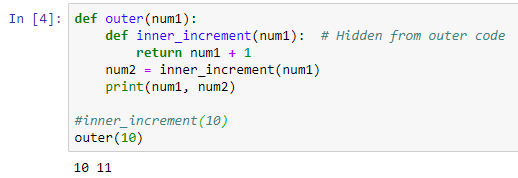Another example,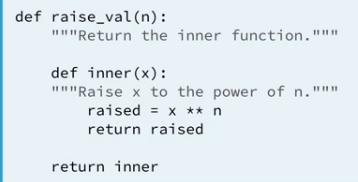When we try to execute this code by calling the raise_val(), we do not need to repeatedly write the codes twice.

```#function call
square = raise_val(2)
cube = raise_val(3)
print(square(2), cube(4))

#output
#4 64
```

I have a question before proceed, how does the line of code works?
print(square(2), cube(4))

While n value (argument) for function raise_val() is 2 and 3 respectively. the variable square and cube pass an argument too.

Keeping it DRY
Maybe, you have a function that performs the same chunk of code in numerous places. DRY means “don’t repeat yourself”. In an example I found online, you might write a function that processes a file, and you want to accept either an open file object or a file name.

The code looks like,

```def process(file_name):
def do_stuff(file_process):
for line in file_process:
print(line)
if isinstance(file_name, str):
with open(file_name, 'r') as f:
do_stuff(f)
else:
do_stuff(file_name)
```
```# Define three_shouts
def three_shouts(word1, word2, word3):
"""Returns a tuple of strings
concatenated with '!!!'."""

# Define inner
def inner(word):
"""Returns a string concatenated with '!!!'."""
return word + '!!!'

# Return a tuple of strings
return (inner(word1), inner(word2), inner(word3))

# Call three_shouts() and print
print(three_shouts('a', 'b', 'c'))

#Output returns a tuple of 3 elements
#('a!!!', 'b!!!', 'c!!!')
```

Remember that assigning names will only create or change local names, unless they are declared in global or nonlocal statements using keywords, global or nonlocal.

The syntax,

```def outer():
n = 1
def inner():
nonlocal n
n = 2
print(n)
inner()
print(n)
```

The above code alters the value of n in the enclosing scope. When outer() function is called, the n = 1 has changes its value by inner() function using the keyword, nonlocal n. Therefore, both print statements return 2.

# Python Scope Rules in FunctionScope Rules in Functions

Functions provide a nested namespace (sometimes called a scope), which localizes the names they use, such that names inside the function will not clash with those outside (in a module or other function). We usually say that functions define a local scope, and modules define a global scope.

The LGB Rule:
– Name references search at most three scopes: local, then global, then built-in.
– Name assignments create or change local names by default.
– “Global” declarations map assigned names to an enclosing module’s scope.

In other words, all names assigned inside a function `def` statement are locals by default; functions can use globals, but they must declare globals to change them.

When you use an unqualified name inside a function, Python searches three scopes—the local (L), then the global (G), and then the built-in (B)—and stops at the first place the name is found.

When you assign a name in a function (instead of just referring to it in an expression), Python always creates or changes the name in the local scope, unless it’s declared to be global in that function.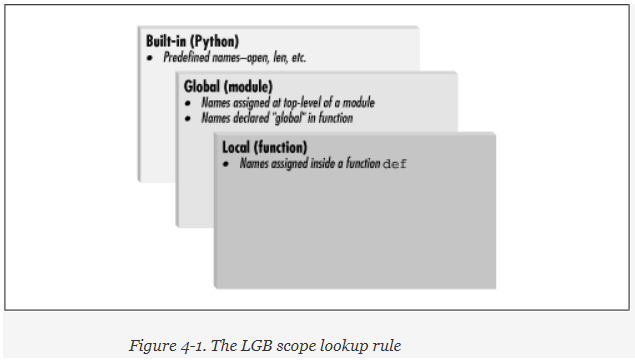An example code is given to further illustrate the concept.

```# global scope
X = 99                # X and func assigned in module: global

def func(Y):          # Y and Z assigned in function: locals
# local scope
Z = X + Y         # X is not assigned, so it's a global
return Z

func(1)               # func in module: result=100
```

X and func are global names because it is assigned at top-level
Y and Z are local names to the function.

## Local scope vs global scope

The first example below shows the local scope, new_val within the function, is not related to the global new_val = 10. The local scope uses the value = 3 to calculate the square of the number. As a result, it returns 9 when function square() is called and global new_val did not change.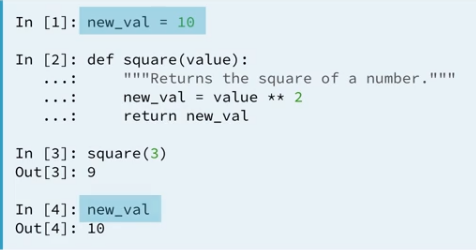In my earlier paragraph, “functions can use globals, but they must declare global to change them. ” Below sample illustrates the meaning of this paragraph where,

```global new_val
```

is declared within the function and now, the new_value = 10 and it calculates the square of the number which returns 100. It does not take the parameter passes in square() function. Here, the new_val has been modified.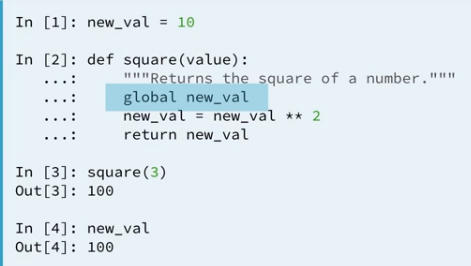And, this looks different that the following sample code, where it takes reference of the global variable new_val = 10 and did not change it within the function after the calculation. It then, reassigns with a new value of new_val = 20 and repeats the function calls.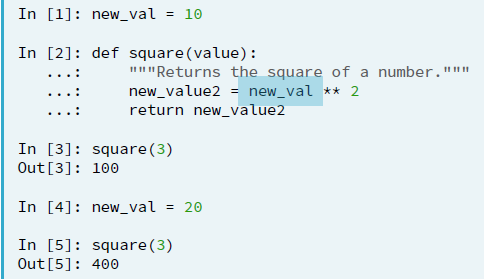## Built-in scope

To use the built-in scope in Python, a module called builtins is needed. After executing the code,
import builtins

we can execute the next code,
dir(builtins)

which print a list of all the names in the module builtins. You can find some familiar names within the list.

Source:
https://www.oreilly.com/library/view/learning-python/1565924649/ch04s03.html

Summary of the day:

• Python’s scope rules
• global scopes
• local scopes
• built-in scopes

# Python: Pig Latin

• If word starts with a vowel, add ‘ay’ to end.
• If word does not start with a vowel, put first letter at the end, then add ‘ay’
• Example:
• word > ordway
• apple > appleay

To further understand and practise the functions in Python, the tutor shared one of the case studies in the online web learning. The case study detail is as above and the workaround is taking the first character and check if it matches the condition.

If yes, then appends the ‘ay’ to the end.
If no, then takes the 2nd character onward, appends the 1st character and follows by appending ‘ay’ to the end.

Sample code as below screenshot: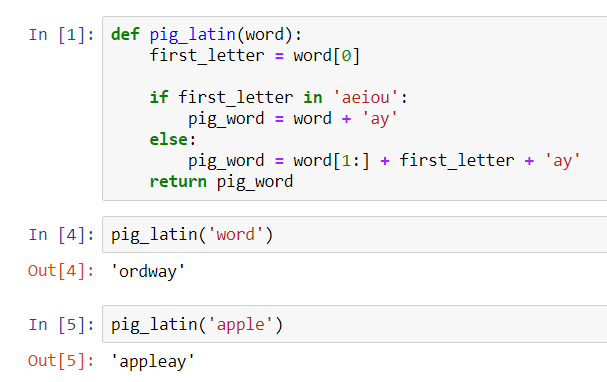# Day 18: Functions in Python

Continuous from the introduction of functions and methods in Python in my previous blog entry. I added a few more examples to understand functions better. In the next examples, I extracted it from DataCamp and Udemy’s online web learning courses.

Built-in Functions
Most of us familiar with len(), str(), format() or print() which I used as examples in previous blog entries. These are built-in Functions from Python which we used to get the length of the string, converting a value to a string and format the value to print out a line accordingly.

Hope, till here, it is simple to go ahead with an example of str() to recap. See below for code snippet and the output from the Jupyter Notebook.

```x = str(10)
print(x)
print(type(x))
```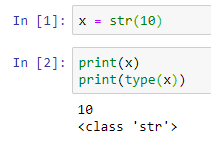It prints out the value 10 as an ‘str’ (string) class. Do you still remember, if we want to print a number, we convert the number to string data type and concatenate with other strings of words (if any) to print out the whole statement? The str() converts number to string. Otherwise, it returns an int data type and it cannot be concatenated with strings.

Now, move on to defining our functions with examples and explanations below. Still remember the keywords for defining a function?

• Keyword def marks the start of function’s header.
• Function name, abc, is uniquely named.
• Parameters (arguments) which will pass values to the function. It is optional. In the example, a and b are the values pass to the function.
Colon (:) marks the end of the function’s header.
• Indentation after the newline.
• Docstring, an optional documentation to describe what the function does, usually helpful to developers.
• Execution statements.
• Return statement to return a value from the function. It is optional.

## Defining a function without a parameter

Let begin to write a simple user defined function with the code snippet below based on the function’s syntax I mentioned above. We choose to leave the parameter as an option, so we do not define it.

```def square():
new_value = 2 ** 2
print(new_value)
print(type(new_value))

square()
```

After writing the function block of codes, we need to call our function. The above function does not do or return anything on the screen unless we do a call or we invoke the function. That is why the next line of code is required:
square()

Upon execution, it prints the value on the screen accordingly. It does not require any input of arguments in this code. And the value is fixated to use,
new_value = 2 ** 2

every time it is being called. it returns the same output.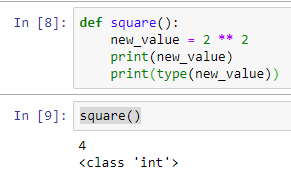## Defining a function with a parameter and provide a default value of a parameter.

How about now, we put in the parameter of our function? How to code it, example below:

```def sayhello(name):
print('hello ' + name)
```

When I call the function sayhello() with an argument, syntax like this,
sayhello(‘Li Yen’)

the output on the screen shows,
hello Li Yen

Up to here, probably, you want to know why sometimes I use parameters and arguments. The DataCamp’s tutorials on Python Data Science Toolbox, gave a good example to example this. The parameter is used when defining a function and we call a function, we pass an argument into the function to be executed. I hope I can make it clear enough and try to distinguish these two jargon.

What if I did not input a value (argument) to the function? The above function expects a value and if we execute it without a value, Python throws an error.

While the parameter defines to accept a value (argument) from the user, it can be set with a default argument by giving it a variable and a value such as name = ‘User’. The variable, name has a value of ‘User’. This is how the code looks like,

```def sayhello(name='User'):
print('hello ' + name)
```

So, when we call the same function again without passing argument, syntax like this,
sayhello()

the output on the screen shows,
hello User

Another example below, showing the combination of using the default value, yet I’m passing my name into the function. It overwrites the value of ‘User’. Cool!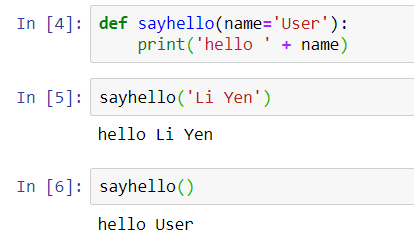## Multiple Function Parameters

Accept more than 1 parameter and when we call a function, number of arguments same as number of parameters. The values are assigned in sequence of the parameters.

Call function: # of arguments = # of parameters. Below is the examples of multiple parameters:

```def raise_to_power(value1, value2):
"""Raise value1 to the power of value2."""
new_value = value1 ** value2
return new_value

result = raise_to_power(2, 3)
print(result)
```
```def square(value1, value2):
new_value = value1 ** value2
return new_value

square(10,3)
```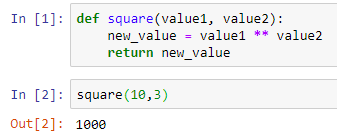Is it straight forward enough with the above example code and output? When the square(10,3) is called, the value of 10 passes to variable, value1 and the value of 3 passes to variable, value2, then the variable new_value holds the value of the computation of value1 ** value2. In syntax format, it looks like this,

```new_value = 10 ** 3
```

To call the square() function, declare a variable result and print the output of result:

```result = square(10,3)
print(result)
```

## Return statement

Return a Boolean from a function
We can return a Boolean from a function, just like other data types too. The example below, I’m returning a Boolean’s value.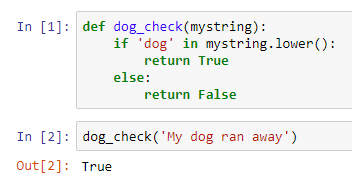If we check the condition as below,

```'dog' in 'My dog ran away'
```

It returns True.

So, in that example, the tutor said, we can simplify the codes by removing the if statement to achieve the same result. It can be done in one line.

```def dog_check(mystring):
return 'dog' in mystring.lower()
```

## Return multiple values

As mentioned in my summary post, the return statement can return more than one value. When I learned it through DataCamp, this can be happened when a function returns a tuple. We covered what tuple is. It is a collection of data, just like a list of parentheses. It is immutable, which means data cannot be changed.
Tuple (‘a’, ‘b’, ‘c’, ‘d’)

Below is the screenshot of the sample code: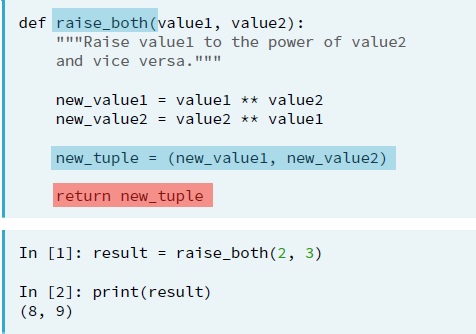Another sample code from DataCamp, an expand from the above square() function to return 2 values in a tuple, unpack the tuple and print out a value using index.

```def square(value1, value2):
new_value1 = value1 ** value2
new_value2 = value2 ** value1

new_tuple = (new_value1, new_value2)
return new_tuple

result = square(10,3)
print(result)
print(result) #print(square(10,3))
print(result) #print(square(10,3))
```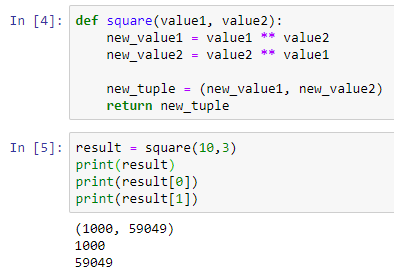The above shows a combination of using functions with multiple parameters, returning the multiple values using a tuple and print out the result by unpacking the tuple and print out the result of new_value1 and new_value2 by its index.

Summary of the day:

• Define function without parameter.
• Define a function with a parameter.
• Use the default argument in the parameter.
• Use the return statement with a single value.
• Use the return statement with tuple for multiple values.
• Unpack tuple and using index slicing to print the value of a tuple.

# Day 18: Methods and Functions in Python

I did some revision before I continued with the new chapter of my learning in Udemy’s Complete Python Bootcamp, From zero to hero. I tried to look for a simpler explanation of the differences between methods and functions in Python.

Trying to put it in a dummy way to explain sometimes, it requires a good example, just like this one I found in Quora.com, written by Sakina Mirza.

A user-defined function.
A function is a block of code to carry out a task and it calls by its name. All functions may have zero or many arguments. The arguments are passed explicitly (directly). On the exit of the function, it may or may not return value or values.

Python allows us to define our own function calls add with a and b as the arguments, just like an example below:

```def add(a,b):
return a + b
```

Main things to remember:

• Keyword def marks the start of function’s header.
• Function name, abc, is uniquely named.
• Parameters (arguments) which will pass values to the function. It is optional. In the example, a and b are the values pass to the function.
• Colon (:) marks the end of the function’s header.
• Indentation after the newline.
• Docstring, an optional documentation to describe what the function does, usually helpful to developers.
• Execution statements.
• Return statement to return a value from the function. It is optional.

How to call the above function in Python?
We can call it from another function, program or the Python prompt. To call a function, simply type the function name with the parameters if specified.

In our example, it types as,

```abc(7, -4)
```

The return statement is used to exit a function and go back to the point where it was called. If a function does not have a return statement, then the function will return the None object. Function ends here and usually, it could be a printout statement to indicate end of a function.

Our return value is 3 for the above.

Other examples of functions are the common ones we used before this, such as len(), str(), format() or even print(). These are the built-in functions in Python and they return values or output when we call it. So, 2 types of functions to be remembered in Python, built-in functions and user defined functions.

Python Methods.
It is like a function, except it is attached to an object (dependent). A method is implicitly (indirectly) passed to the object for which it is invoked. It may or may not return a value or values. The method is accessible to data that is contained within the class.

This means we call a method (which is a function belongs) on (to) an object and it possibly makes changes to that object. Let take an example below:

```class vehicle:
def __init__(self, color):
self.color = color
def start(self):
print("Starting engine")
def showcolor(self):
print(f"I am {self.color}")
```

Main things to remember:

• Keyword class marks the start of the header.
• Class name, vehicle, is uniquely named.
• Colon (:) marks the end of the function’s header.
• Indentation after the newline.

How to call a method?
We create an object called, car and we can use the class named, vehicle. Noticed, the example used,
__init__() function

It is an built-in function in Python. All classes have a function called __init__() which is executed when the class is being initiated. Use it to assign values to object properties or other operations that are necessary to do when the object is being created.

So, that explained the first part of the codes above when we assign ‘black’ to the object ‘car’.

```car = vehicle('black')
car.start()
car.showcolor()
```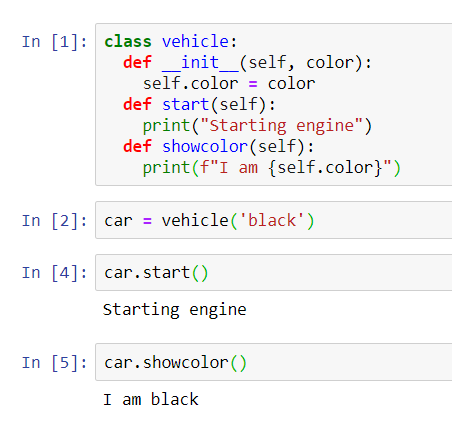When we called the functions, start() and showcolor() from the object, car, the print out the statements as shown above. I will share a bit more examples I extracted from the online web learning course in my next blog.

Summary of the day:

• User-defined function and built-in function.
• Method, class and object.